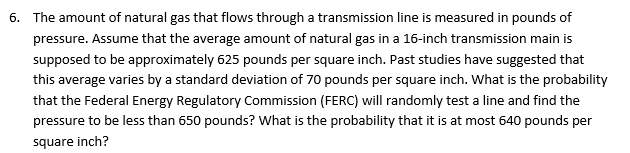The amount of natural gas that flows through a transmission line is measured in pounds ofpressure. Assume that the average amount of natural gas in a 16-inch transmission main issupposed to be approximately 625 pounds per square inch. Past studies have suggested thatthis average varies by a standard deviation of 70 pounds per square inch. What is the probabilitythat the Federal Energy Regulatory Commission (FERC) will randomly test a line and find thepressure to be less than 650 pounds? What is the probability that it is at most 640 pounds persquare inch?6.

Questionhelp_outlineImage TranscriptioncloseThe amount of natural gas that flows through a transmission line is measured in pounds of pressure. Assume that the average amount of natural gas in a 16-inch transmission main is supposed to be approximately 625 pounds per square inch. Past studies have suggested that this average varies by a standard deviation of 70 pounds per square inch. What is the probability that the Federal Energy Regulatory Commission (FERC) will randomly test a line and find the pressure to be less than 650 pounds? What is the probability that it is at most 640 pounds per square inch? 6. fullscreen
Step 1

Given

X~N(625,70)

Note: 70 is standard deviation

We have used the method to standardize the normal distribution to make it standard normal distribution to get the required probability. Formula to standardize the normal distribution is given on white board.

Step 2

In this part we have to find the probability that the federal regulatory commission will randomly test a line and find the pressure to be less than 650 pound...

Want to see the full answer?

See Solution

Want to see this answer and more?

Our solutions are written by experts, many with advanced degrees, and available 24/7

See Solution
Tagged in

Other